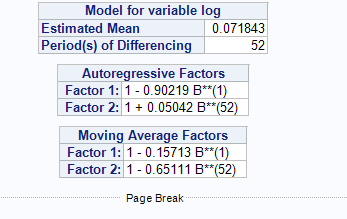## description of SARIMA model

I have built a proper model through the Arima process step, but I don't know how to formally and correctly describe the model.this is my final arima model,it should name "ARIMA(1,1,1)(1,1,1)52" or another???

1 ACCEPTED SOLUTION

Accepted Solutions

## Re: description of SARIMA model

Hello @Phoenix_LJ ,

I am assuming you are familiar with the backshift notation and polynomials in the backshift operator.

Here's the formulae of your ARIMA(1,0,1)(1,1,1)52 model :

• Z_t =  ( 1 - B ) ( 1 - B**52 ) Y_t
• ( 1 - 0.90219B ) ( 1 + 0.05042B**52) Z_t = 0.071843 + (1 - 0.15713B ) ( 1 - 0.65111B**52 ) e_t

This is indeed a SARIMA model, i.e. Seasonal ARIMA (Auto-Regressive Integrated Moving Average) model with :

• seasonal differencing (once on 52 periods = simple seasonal differencing). The degree d of the seasonal differencing is 1 and s=52 is the length of the seasonal cycle.
• 1 is the order of the autoregressive part
• 1 is the order of the seasonal autoregressive part
• 1 is the order of the moving-average process
• 1 is the order of the seasonal moving-average process
• e_t is the independent disturbance, also called the random error
• you have no transfer functions as it is not SARIMAX (with some extra X-input time series)

Kind regards,

Koen

2 REPLIES 2

## Re: description of SARIMA model

Hello @Phoenix_LJ ,

I am assuming you are familiar with the backshift notation and polynomials in the backshift operator.

Here's the formulae of your ARIMA(1,0,1)(1,1,1)52 model :

• Z_t =  ( 1 - B ) ( 1 - B**52 ) Y_t
• ( 1 - 0.90219B ) ( 1 + 0.05042B**52) Z_t = 0.071843 + (1 - 0.15713B ) ( 1 - 0.65111B**52 ) e_t

This is indeed a SARIMA model, i.e. Seasonal ARIMA (Auto-Regressive Integrated Moving Average) model with :

• seasonal differencing (once on 52 periods = simple seasonal differencing). The degree d of the seasonal differencing is 1 and s=52 is the length of the seasonal cycle.
• 1 is the order of the autoregressive part
• 1 is the order of the seasonal autoregressive part
• 1 is the order of the moving-average process
• 1 is the order of the seasonal moving-average process
• e_t is the independent disturbance, also called the random error
• you have no transfer functions as it is not SARIMAX (with some extra X-input time series)

Kind regards,

Koen

## Re: description of SARIMA model

• Thank you very much for your help!

Discussion stats
• 2 replies
• 482 views
• 1 like
• 2 in conversation